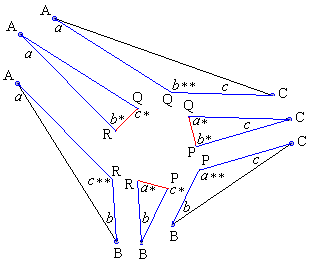## Morley's MiracleRemarks on J. Conway's proof

As other "backward" proofs, Conway's ends up with a triangle that at best is similar to ΔABC which is of course fine.The seven triangles fit together for two reasons:

1. At the vertices of the equilateral triangle the angles sum up to 360°.
2. The line segments that are to be common sides of two triangles are equal by construction. This is clear for the sides of the equilateral triangle that have been chosen to be 1. The add-on points Y and Z allow to select triangles BPC, AQC, and ARB so that the potential trisectors also match each other.

The whole point of introducing points Y and Z is to eschew trigonometry. Building on the rigidity of the configuration and the fact that all angles in all triangles are known, it is possible to follow the chain of six triangles (the middle one excluded) using the Law of Sines:Start, for example with ΔABR. Fix, say, AR. Then

 (1) BR = AR·sin(a)/sin(b).

Construct ΔBPR with BR given above. Then

 (2) BP = BR·sin(a*)/sin(c*).

Keep constructing triangles so that their sides match. By the Law of Sines, we'll sequentially get

 (3) CP = BP·sin(b)/sin(c) (4) CQ = CP·sin(b*)/sin(a*) (5) AQ = CQ·sin(c)/sin(a) (6) AR = AQ·sin(c*)/sin(b*)

The last one should actually read "AR' = AQ·sin(c*)/sin(b*)". For we do not know in advance whether it has the same length as AR. To see that it does, multiply (1)-(6): after reducing like factors we end up with AR' = AR. Therefore, putting together the six triangles fills a triangle with a triangular hole. Counting angles we see that the hole is equiangular. Which is what was needed.

One should appreciate the simplicity of Conway's proof that not only avoids the trigonometry but makes it also unnecessary to handle all 6 triangles separately. D.J.Newman's proof is a simplified trigonometric variant where only 3 (out of 6) triangles are handled with the Law of Sines.### Morley's Miracle

#### Invalid proofs